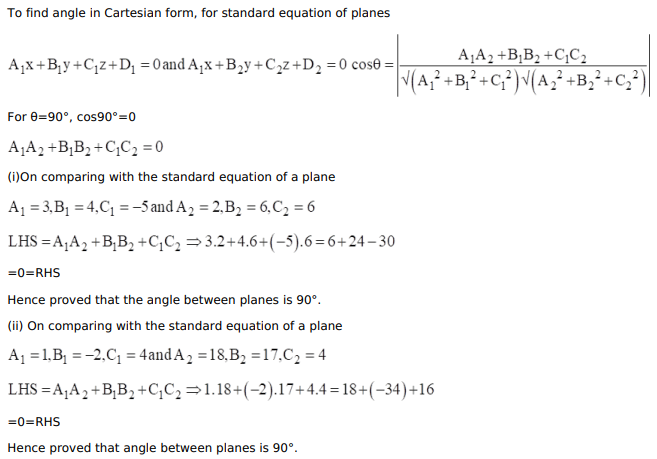# Show that each of the following pairs of planes are at right angles:

Question:

Show that each of the following pairs of planes are at right angles:

(i) $3 x+4 y-5 z=7$ and $2 x+6 y+6 z+7=0$

(ii) $x-2 y+4 z=10$ and $18 x+17 y+4 z=49$

Solution: